Categoricals are a way of representing columns of data, that provide:

• categories: a custom ordering to use when sorting the values.
• codes: a compressed representation of the data as integers.

While codes were historically important for representing large columns of data, the big value of categoricals today is as a tool for customizing order in plots. This might seem like a small job, but as it turns out it is very important in data analysis.

This page will discuss the `pandas.Categorical` class for creating categoricals, as well as a helper submodule `siuba.dply.forcats` with helper functions for working with this kind of data.

``````import pandas as pd

from siuba import _, count, mutate

from siuba.dply.forcats import fct_inorder, fct_infreq, fct_reorder, fct_lump
from siuba.data import penguins``````

## Required datasets

This page uses the `nycflights13` dataset, which can be installed using pip:

``pip install nycflights13``

## Overview

Here is a simple `pd.Categorical` representing a column with 3 values.

``````x = ["front", "middle", "back"]

a_cat = pd.Categorical(x)
a_cat``````
``````['front', 'middle', 'back']
Categories (3, object): ['back', 'front', 'middle']``````

Notice that the bottom line of the print out shows the categories ordered as `['back', 'front', 'middle']`. By default, `pd.Categorical` categories are in (roughly) alphabetical order. Ideally, we’d have them in an order like front, middle, back!

One way to do this, is to use `fct_inorder()` to order by first observed first, second observed second, etc..

``fct_inorder(x)``
``````['front', 'middle', 'back']
Categories (3, object): ['front', 'middle', 'back']``````

The remaining sections will focus on two kinds of categorical helper functions:

• functions for ordering category levels.
• functions for grouping categories together.

However, before we do that, let’s go through a few useful ways to interact with categoricals.

### Core attributes

``a_cat.codes``
``array([1, 2, 0], dtype=int8)``
``a_cat.categories``
``Index(['back', 'front', 'middle'], dtype='object')``
``a_cat.ordered``
``False``

### Wrapping in `pd.Series`

pandas often wraps categoricals in a Series object.

``````a_cat2 = pd.Categorical(["b", "a", "c"])
ser = pd.Series(a_cat2)
ser``````
``````0    b
1    a
2    c
dtype: category
Categories (3, object): ['a', 'b', 'c']``````

For example, any time you create a DataFrame column out of a categorical, it gets wrapped in a pd.Series.

``````df = pd.DataFrame({"some_cat": a_cat2})

print(type(df.some_cat))
df.some_cat``````
``<class 'pandas.core.series.Series'>``
``````0    b
1    a
2    c
Name: some_cat, dtype: category
Categories (3, object): ['a', 'b', 'c']``````
Note

99% of the time when doing data analysis, your categorical is wrapped in a Series.

Note that accessor methods like `.str.upper()` are available on the series, and the underlying category attributes are availble using the `.cat` accessor.

``ser.str.upper()``
``````0    B
1    A
2    C
dtype: object``````
``ser.cat.codes``
``````0    1
1    0
2    2
dtype: int8``````

You can get the underlying categorical out using the `.array` property.

``ser.array``
``````['b', 'a', 'c']
Categories (3, object): ['a', 'b', 'c']``````

### Using in verbs

The functions in `siuba.dplyr.forcats` can be used with lazy expressions.

``fct_inorder(_.species)``
``````█─'__call__'
├─█─'__custom_func__'
│ └─<function fct_inorder at 0x7fb0cff61a60>
└─█─.
├─_
└─'species'``````

Note how the above output is a lazy expression, which can be used inside verbs like `mutate()`:

``````(penguins
>> mutate(
species_cat = fct_inorder(_.species),
species_cat2 = _.species.astype("category"),
)
)``````
species island bill_length_mm bill_depth_mm flipper_length_mm body_mass_g sex year species_cat species_cat2
... ... ... ... ... ... ... ... ... ... ...
342 Chinstrap Dream 50.8 19.0 210.0 4100.0 male 2009 Chinstrap Chinstrap
343 Chinstrap Dream 50.2 18.7 198.0 3775.0 female 2009 Chinstrap Chinstrap

344 rows × 10 columns

## Order categories by counts with `fct_infreq()`

Use `fct_infreq` to order category levels by their frequency in the data.

``fct_infreq(penguins.species)``
``````0         Adelie
...
342    Chinstrap
343    Chinstrap
Length: 344, dtype: category
Categories (3, object): ['Adelie', 'Gentoo', 'Chinstrap']``````

In the output above, the category ordering shows us that “Adelie” is most frequent in the data, followed by “Gentoo”, and then “Chinstrap”.

We can verify this explicitly by using the verb `count()` to tally up each species.

``````tbl_species_count = penguins >> count(_.species)

tbl_species_count``````
species n
1 Chinstrap 68
2 Gentoo 124

Ordering by frequency is helpful for giving viewers a rough sense for which groups have less data in your plots.

For example, the code below plots each species on the x-axis, bill_depth_mm on the y-axis. It orders the categories of species by frequency, so those with the most data are shown on the left.

``````from plotnine import ggplot, aes, geom_point, position_jitter

(penguins
>> mutate(species = fct_infreq(_.species))
>> ggplot(aes("species", "bill_depth_mm"))
+ geom_point(position=position_jitter(width=.1, height=0))
)``````
``/opt/hostedtoolcache/Python/3.9.16/x64/lib/python3.9/site-packages/plotnine/layer.py:411: PlotnineWarning: geom_point : Removed 2 rows containing missing values.``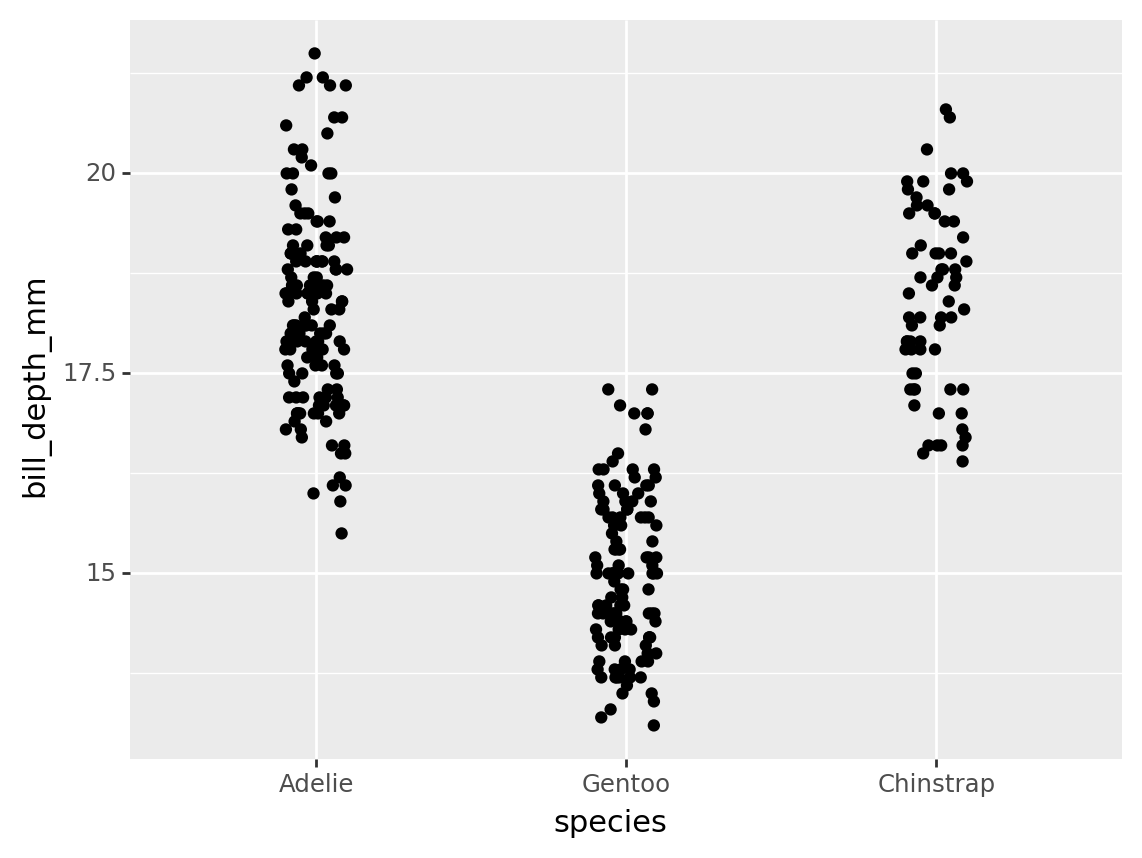``<ggplot: (8774818531639)>``

Note that the `position_jitter(width=.1, height=0)` tells the plot to randomly adjust the width of each point between `+-.1` (where the distance between each species label is 1).

## General reordering with `fct_reorder()`

Use `fct_reorder()` to reorder the categories of a column, based on another column.

This function takes 3 main arguments:

• A column to copy and return with reordered categories.
• A column used to calculate the new ordering.
• An optional function that performs a calculation (defaults to calculating the median).

For example, the code below reorders the categories of the `species` column.

``fct_reorder(penguins.species, penguins.bill_depth_mm, "mean")``
``````0         Adelie
...
342    Chinstrap
343    Chinstrap
Length: 344, dtype: category
Categories (3, object): ['Gentoo', 'Adelie', 'Chinstrap']``````

Note that it reorders `species` based on the mean of `bill_depth_mm` within each category.

Note

Currently, the easiest way to specify a calculation is by passing a string, like `"mean"`. Under the hood, `fct_reorder()` calls `pd.Series.agg()`, so you could also pass a lambda or function directly.

### Basic example

The code below reorders `species` using the default function (“median”) over `bill_depth_mm`. This results in boxplots are ordered from lowest to highest median.

``````from plotnine import ggplot, aes, geom_boxplot

(penguins
>> mutate(species = fct_reorder(_.species, _.bill_depth_mm))
>> ggplot(aes("species", "bill_depth_mm"))
+ geom_boxplot()
)``````
``/opt/hostedtoolcache/Python/3.9.16/x64/lib/python3.9/site-packages/plotnine/layer.py:333: PlotnineWarning: stat_boxplot : Removed 2 rows containing non-finite values.``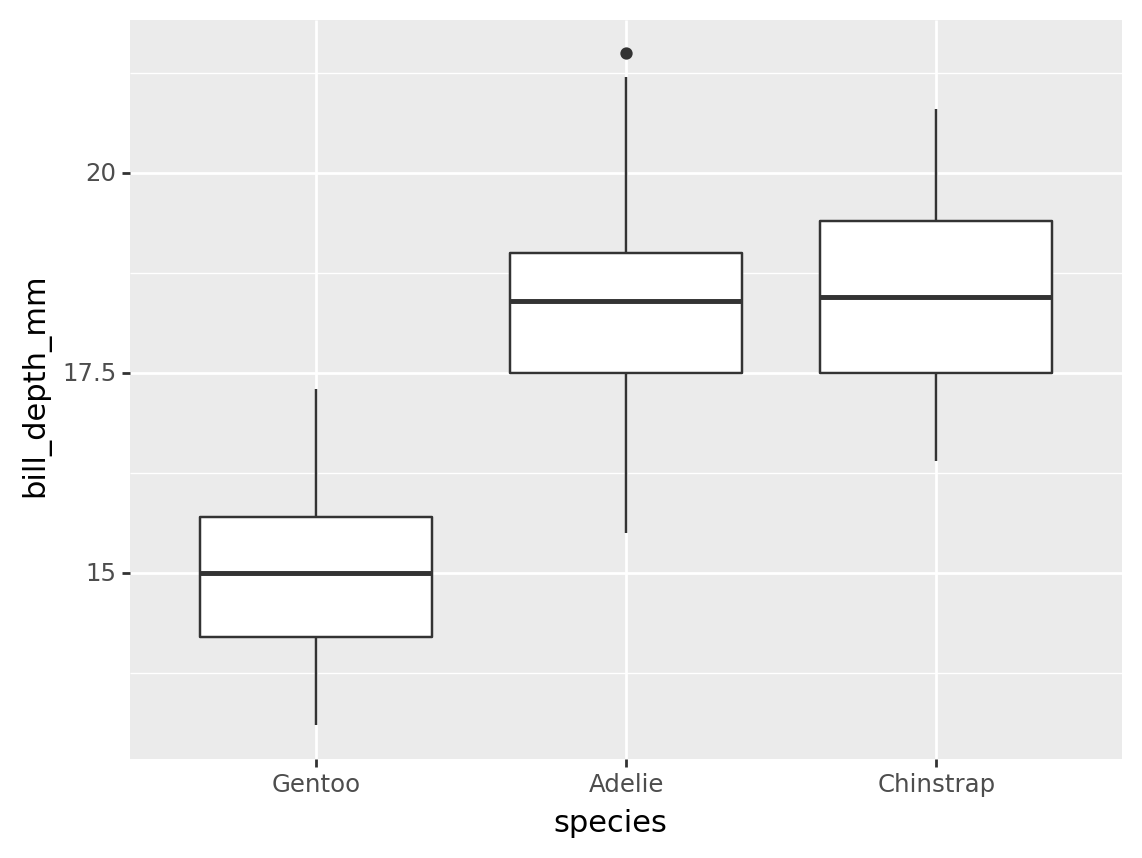``<ggplot: (8774815514989)>``

### Used with count

A common use for `fct_reorder` is to reorder a rolled up count.

For example, the code below counts the number of rows per species.

``````tbl_penguin_species = penguins >> count(_.species)
tbl_penguin_species``````
species n
1 Chinstrap 68
2 Gentoo 124

Suppose we had a table like this one, we might want to reorder the categories based on the `n` column.

``fct_reorder(tbl_penguin_species.species, tbl_penguin_species.n, desc=True)``
``````0       Adelie
1    Chinstrap
2       Gentoo
dtype: category
Categories (3, object): ['Adelie', 'Gentoo', 'Chinstrap']``````

Note that above we used the `desc=True` argument to put the categories in descending order. Because there is only entry per category level, the default function (“median”) just returns that value or `n`. This results in categories ordered by `n`.

Here is the same calculation used to reorder the bars on a plot.

``````from plotnine import ggplot, aes, geom_col

(tbl_penguin_species
>> mutate(species = fct_reorder(_.species, _.n, desc=True))
>> ggplot(aes("species", "n"))
+ geom_col()
)``````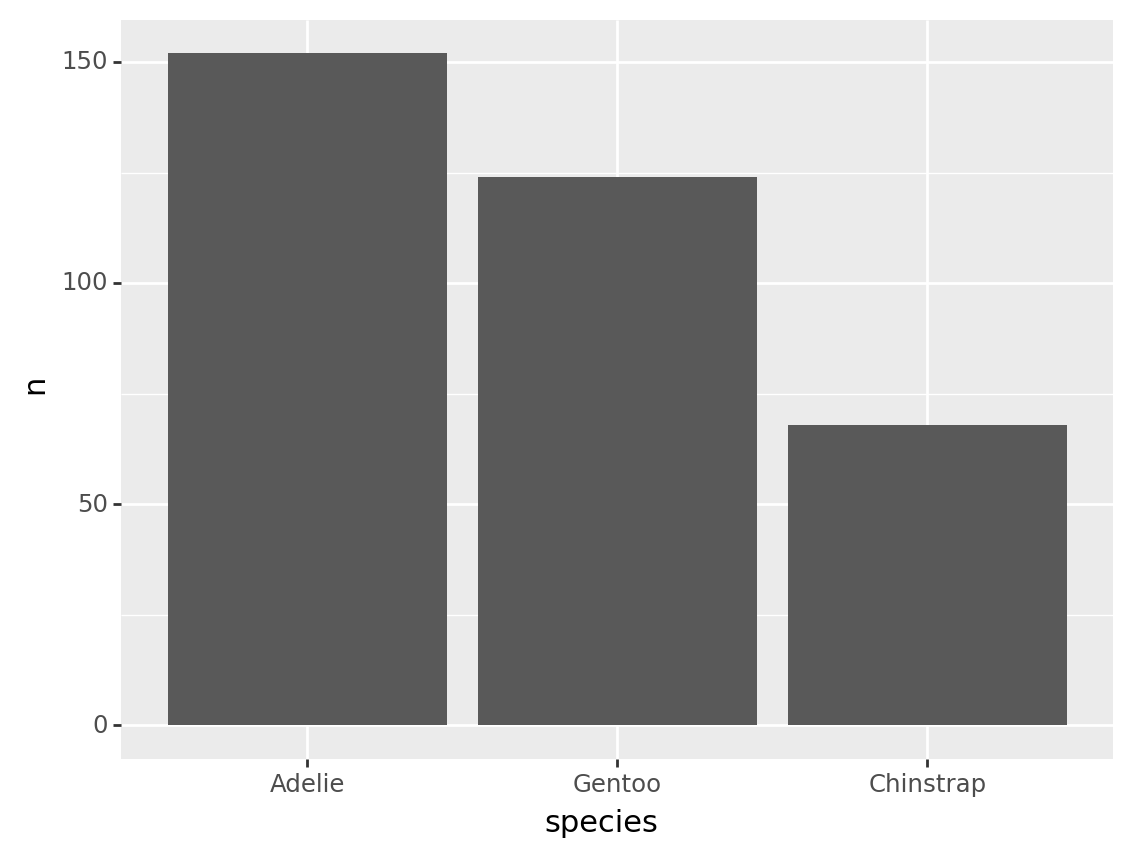``<ggplot: (8774818395525)>``

## Binning categories with `fct_lump()`

While functions like `fct_infreq()` and `fct_reorder()` change the order of categories, functions like `fct_lump()` reduce the number of categories.

Use `fct_lump()` to lump categories with fewer observations into a single category (e.g. “Other”).

### Basic example

For example, let’s look at the `nycflights13` table `flights`.

``````from nycflights13 import flights

flights``````
year month day dep_time sched_dep_time dep_delay arr_time sched_arr_time arr_delay carrier flight tailnum origin dest air_time distance hour minute time_hour
0 2013 1 1 517.0 515 2.0 830.0 819 11.0 UA 1545 N14228 EWR IAH 227.0 1400 5 15 2013-01-01T10:00:00Z
1 2013 1 1 533.0 529 4.0 850.0 830 20.0 UA 1714 N24211 LGA IAH 227.0 1416 5 29 2013-01-01T10:00:00Z
... ... ... ... ... ... ... ... ... ... ... ... ... ... ... ... ... ... ... ...
336774 2013 9 30 NaN 1159 NaN NaN 1344 NaN MQ 3572 N511MQ LGA CLE NaN 419 11 59 2013-09-30T15:00:00Z
336775 2013 9 30 NaN 840 NaN NaN 1020 NaN MQ 3531 N839MQ LGA RDU NaN 431 8 40 2013-09-30T12:00:00Z

336776 rows × 19 columns

This table has a column for `carrier` that lists each agency running flights. We can use the verb `count()` to quickly see how many unique carriers there are, and get a feel for how many flights each has run.

``````tbl_carrier_counts = flights >> count(_.carrier, sort=True)
tbl_carrier_counts``````
carrier n
0 UA 58665
1 B6 54635
... ... ...
14 HA 342
15 OO 32

16 rows × 2 columns

Notice two pieces:

• There are 16 rows, so 16 carriers
• There is a big difference between the number of `"UA"` and `"OO"` flights (58,665 vs 32).

Let’s use `fct_lump()` to keep only the 7 biggest carriers, and relable the rest to “Other”.

``fct_lump(tbl_carrier_counts.carrier, w=tbl_carrier_counts.n, n=7)``
``````0        UA
1        B6
...
14    Other
15    Other
Length: 16, dtype: category
Categories (8, object): ['AA', 'B6', 'DL', 'EV', 'MQ', 'UA', 'US', 'Other']``````

In the code above, we told `fct_lump()` to lump categories for `carrier`, weighted by the `n` column, and resulting in `n=7` of the original groups.

Here’s an example using the above code to order a barchart.

``````from plotnine import ggplot, aes, geom_col

(tbl_carrier_counts
>> mutate(binned = fct_lump(_.carrier, w=_.n, n=7))
>> ggplot(aes("binned", "n", fill="carrier"))
+ geom_col()
)``````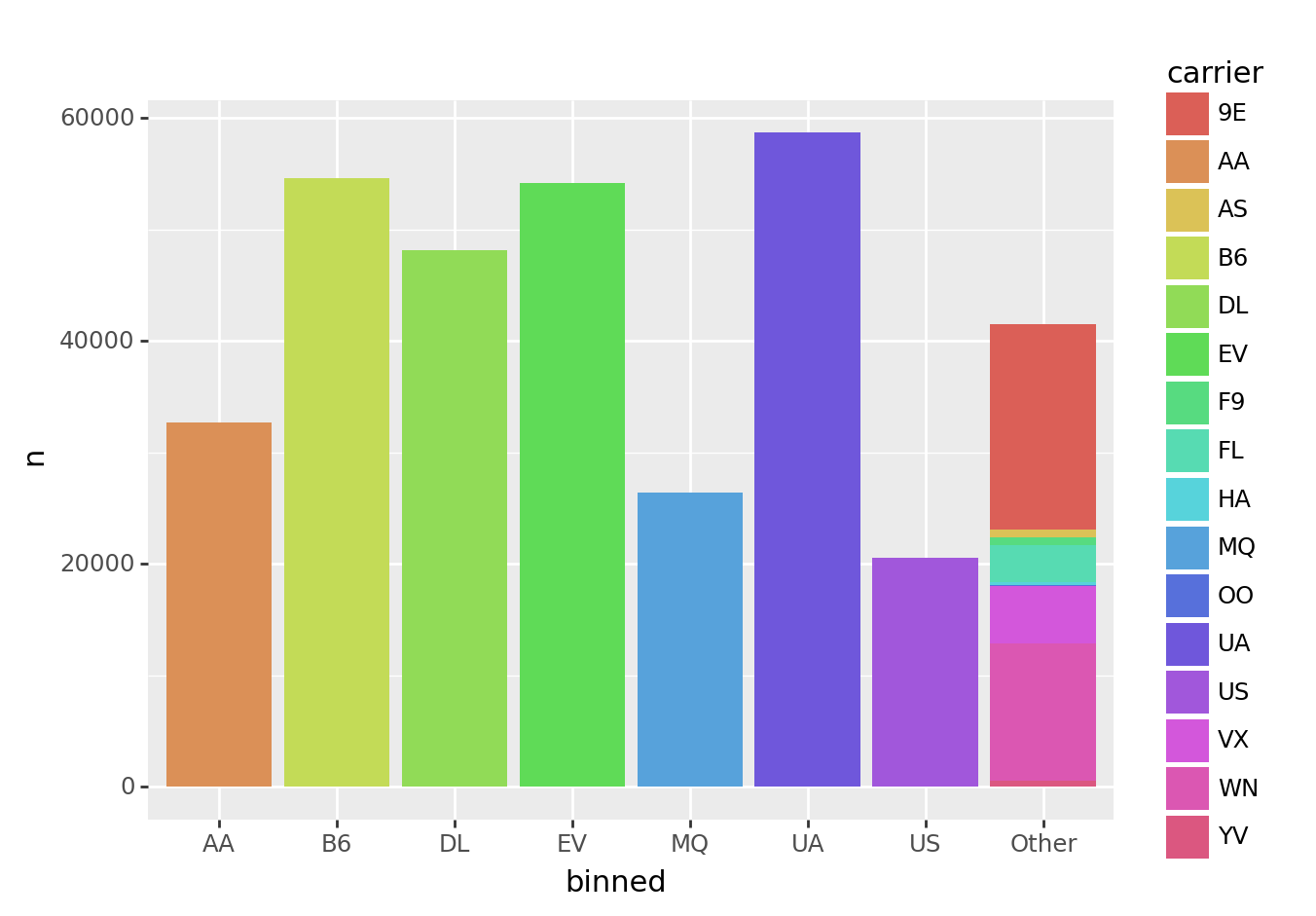``<ggplot: (8774818454971)>``

Notice that all of the smaller carriers are grouped into the “Other” bar.

This plot looks okay, but there are two limitations:

• The first bar on the left is “AA”, but the color legend is in alphabetical order, so starts with “9E”. It would be nice if the legend were in the same order as the bars.
• The bars themselves are not ordered by frequency.

We’ll tackle these pieces in the section below.

### Respecting category order

`fct_lump()` preserves existing category order. This enables you to order categories before collapsing them down.

``````from plotnine import ggplot, aes, geom_col

(tbl_carrier_counts
>> mutate(carrier = fct_inorder(_.carrier))
>> mutate(binned = fct_lump(_.carrier, w=_.n, n=7))
>> ggplot(aes("binned", "n", fill="carrier"))
+ geom_col()
)``````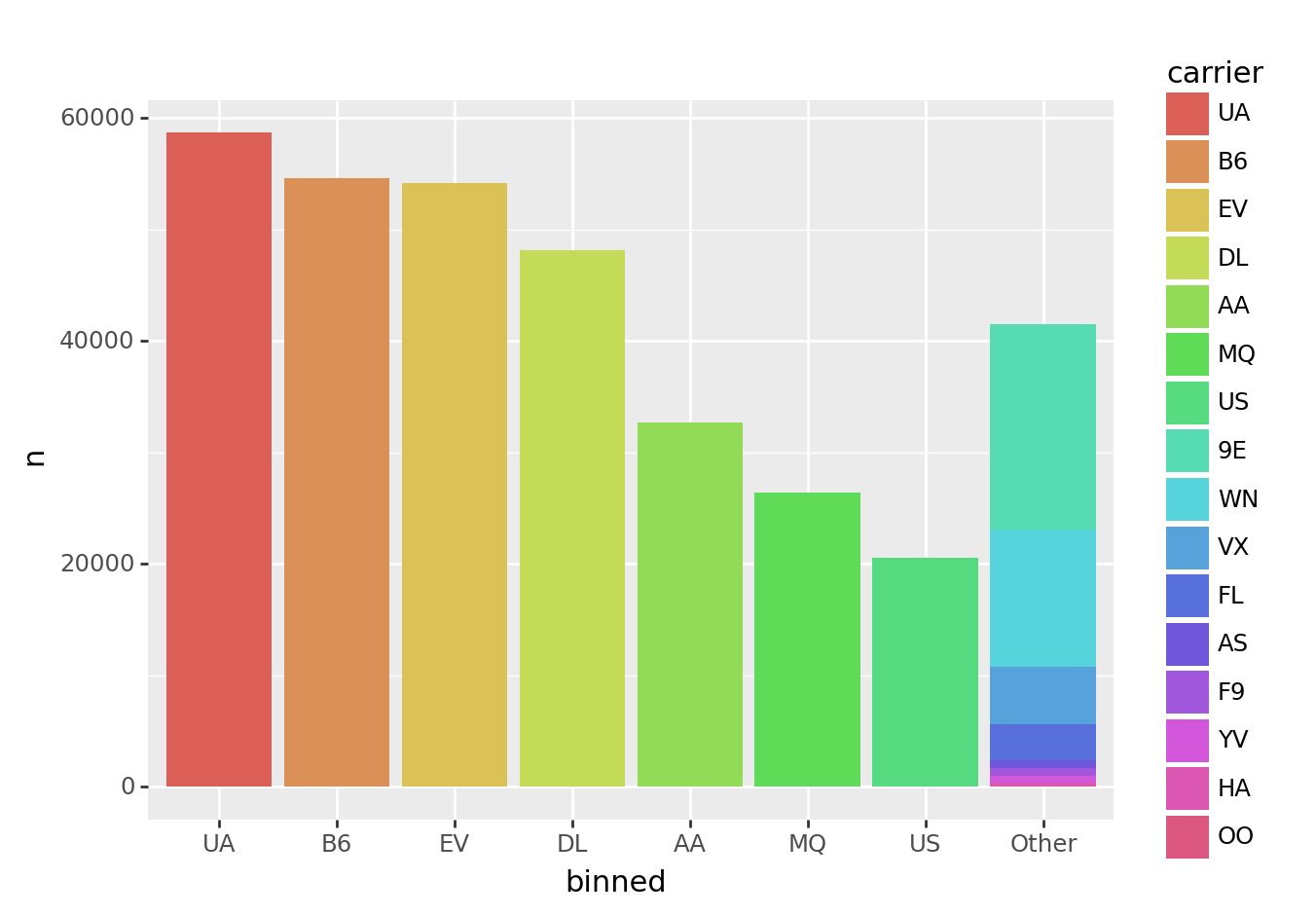``<ggplot: (8774815701523)>``